Courses

Test: Playing With Numbers

10 Questions MCQ Test Mathematics (Maths) Class 8 | Test: Playing With Numbers

Description
Attempt Test: Playing With Numbers | 10 questions in 10 minutes | Mock test for CAT preparation | Free important questions MCQ to study Mathematics (Maths) Class 8 for CAT Exam | Download free PDF with solutions
QUESTION: 1

Substitute digits for the letter A to make the following Addition problem true.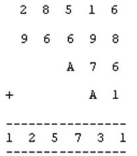Solution:

Explanation : 1+9+7 +4 so the answer will 23 and if we add 5+6+4 so the answer will 17.

QUESTION: 2

Substitute digits for the letter N to make the following Addition problem true.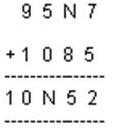Solution:

We have 7+5=12, 1 is carried forward, so 1+N+8=_5, which gives N=6

QUESTION: 3

Substitute digits for the letter A to make the following Addition problem true.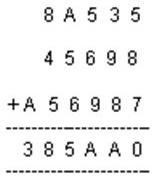Solution:

Adding one’s place 5+7+8=20, 2 is carried forward.adding the ten’s place 2+3+9+8=22, this means A=2

QUESTION: 4

Substitute digits for the letter A to make the following Multiplication problem true.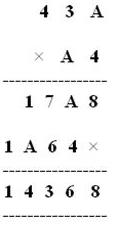Solution:

We have A*4=_8 so A can be 2 or 7.now if A is 7,then we have 2 as carried forward so 4*3+2=14_7A,when A=2, we have no carried forward so4*3=12=_2=A. Hence A=2

QUESTION: 5

Substitute digits for the letters P and Q to make the following Multiplication problem true.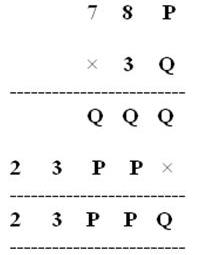Solution:

As we see the addition part,we have 23PPQ, this means that we don’t have any carried forward for the thousand’s place this means we have only single digit by adding Q and P, so Q+P =P only when Q=0,and now seeing the multiplication we have 3*P=_P which is only possible when P=5.

QUESTION: 6

By which of the following number 168 is divisible?

Solution:

The numbers ending with 2,4,6,8 ,0 are divisible by 2.

QUESTION: 7

Substitute digits for the letters A and B to make the following Multiplication problem true.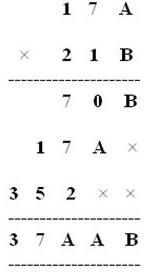Solution:

Looking at the addition of the hundred’s place we have 7+7+2=_A which gives A=6. Coming back to the multiplication which is A*B=B where A=6 ,so B can be 4 or 6, but when you take B=6, we have 3 as carried forward and 7*6+3 is not equal to 0. Hence B=4 and A=6.

QUESTION: 8

By which of the following number 225 is divisible? 2, 3, 4, and 6

Solution:
QUESTION: 9

Suppose that the division N ÷ 5 leaves a remainder of 4, and the division N ÷ 2 leaves a remainder of 1. What must be the one’s digit of N?

Solution:

Since N leaves the remainder of 4 when divided by 5. the possible values in ones place of number N are 4 or 9.

now, since it leaves a remainder of 1 when divided by 2, the N would be an odd number. hence ones digit of N is also an odd number. which means ones digit of our number N is 9.

QUESTION: 10

If a number is divisible by 9, then it is also divisible by which number?

Solution: Yes correct answer is 3 because 9 is divisible by 3 ... 3×3=9..Use Code STAYHOME200 and get INR 200 additional OFF Use Coupon Code

How to Prepare for CAT

Read our guide to prepare for CAT which is created by Toppers & the best Teachers

Track your progress, build streaks, highlight & save important lessons and more!

Similar Content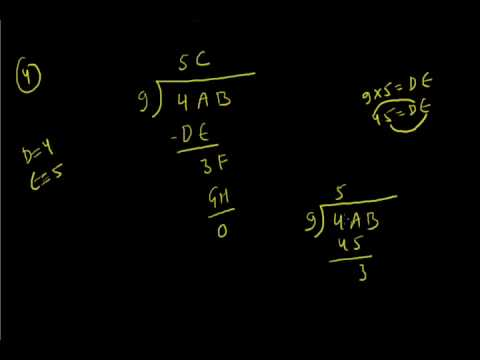Related tests